# Data Organization

The purpose of the devices we investigate is to process digital data, so we will cover some of the basics of data in this section. We will look at binary and hexadecimal numbers, data sizes, endian ordering, and data structures. These concepts are essential to how data are stored. If you have done programming before, this should be a review.

First, let's look at number formats. Humans are used to working with decimal numbers, but computers use binary, which means that there are only 0s and 1s. Each 0 or 1 is called a bit, and bits are organized into groups of 8 called bytes. Binary numbers are similar to decimal numbers except that decimal numbers have 10 different symbols (0 to 9) instead of only 2.

Before we dive into binary, we need to consider what a decimal number is. A decimal number is a series of symbols, and each symbol has a value. The symbol in the right-most column has a value of 1, and the next column to the left has a value of 10. Each column has a value that is 10 times as much as the previous column. For example, the second column from the right has a value of 10, the third has 100, the fourth has 1,000, and so on. Consider the decimal number 35,812. We can calculate the decimal value of this number by multiplying the symbol in each column with the column's value and adding the products. We can see this in Figure 2.1. The result is not surprising because we are converting a decimal number to its decimal value. We will use this general process, though, to determine the decimal value of non-decimal numbers.

Figure 2.1. The values of each symbol in a decimal number.The right-most column is called the least significant symbol, and the left-most column is called the most significant symbol. With the number 35,812, the 3 is the most significant symbol, and the 2 is the least significant symbol.

Now let's look at binary numbers. A binary number has only two symbols (0 and 1), and each column has a decimal value that is two times as much as the previous column. Therefore, the right-most column has a decimal value of 1, the second column from the right has a decimal value of 2, the third column's decimal value is 4, the fourth column's decimal value is 8, and so on. To calculate the decimal value of a binary number, we simply add the value of each column multiplied by the value in it. We can see this in Figure 2.2 for the binary number 1001 0011. We see that its decimal value is 147.

Figure 2.2. Converting a binary number to its decimal value.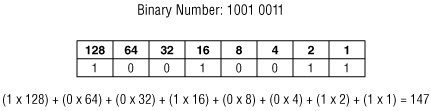For reference, Table 2.1 shows the decimal value of the first 16 binary numbers. It also shows the hexadecimal values, which we will examine next.

Table 2.1. Binary, decimal, and hexadecimal conversion table.

Binary

Decimal

0000

00

0

0001

01

1

0010

02

2

0011

03

3

0100

04

4

0101

05

5

0110

06

6

0111

07

7

1000

08

8

1001

09

9

1010

10

A

1011

11

B

1100

12

C

1101

13

D

1110

14

E

1111

15

F

Now let's look at a hexadecimal number, which has 16 symbols (the numbers 0 to 9 followed by the letters A to F). Refer back to Table 2.1 to see the conversion between the base hexadecimal symbols and decimal symbols. We care about hexadecimal numbers because it's easy to convert between binary and hexadecimal, and they are frequently used when looking at raw data. I will precede a hexadecimal number with '0x' to differentiate it from a decimal number.

We rarely need to convert a hexadecimal number to its decimal value by hand, but I will go through the process once. The decimal value of each column in a hexadecimal number increases by a factor of 16. Therefore, the decimal value of the first column is 1, the second column has a decimal value of 16, and the third column has a decimal value of 256. To convert, we simply add the result from multiplying the column's value with the symbol in it. Figure 2.3 shows the conversion of the hexadecimal number 0x8BE4 to a decimal number.

Figure 2.3. Converting a hexadecimal value to its decimal value.Lastly, let's convert between hexadecimal and binary. This is much easier because it requires only lookups. If we have a hexadecimal number and want the binary value, we look up each hexadecimal symbol in Table 2.1 and replace it with the equivalent 4 bits. Similarly, to convert a binary value to a hexadecimal value, we organize the bits into groups of 4 and then look up the equivalent hexadecimal symbol. That is all it takes. We can see this in Figure 2.4 where we convert a binary number to hexadecimal and the other way around.

Figure 2.4. Converting between binary and hexadecimal requires only lookups from Table 2.1.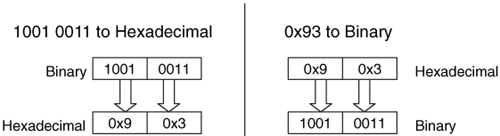Sometimes, we want to know the maximum value that can be represented with a certain number of columns. We do this by raising the number of symbols in each column by the number of columns and subtract 1. We subtract 1 because we need to take the 0 value into account. For example, with a binary number we raise 2 to the number of bits in the value and subtract 1. Therefore, a 32-bit value has a maximum decimal value of

232 - 1 = 4,294,967,295

Fortunately, most computers and low-level editing tools have a calculator that converts between binary, decimal, and hexadecimal, so you do not need to memorize these techniques. In this book, the on-disk data are given in hexadecimal, and I will convert the important values to decimal and provide both.

Data Sizes

To store digital data, we need to allocate a location on a storage device. You can think of this like the paper forms where you need to enter each character in your name and address in little boxes. The name and address fields have allocated space on the page for the characters in your name. With digital data, bytes on a disk or in memory are allocated for the bytes in a specific value.

A byte is the smallest amount of space that is typically allocated to data. A byte can hold only 256 values, so bytes are grouped together to store larger numbers. Typical sizes include 2, 4, or 8 bytes. Computers differ in how they organize multiple-byte values. Some of them use big-endian ordering and put the most significant byte of the number in the first storage byte, and others use little-endian ordering and put the least significant byte of the number in the first storage byte. Recall that the most significant byte is the byte with the most value (the left-most byte), and the least significant byte is the byte with the least value (the right-most byte).

Figure 2.5 shows a 4-byte value that is stored in both little and big endian ordering. The value has been allocated a 4-byte slot that starts in byte 80 and ends in byte 83. When we examine the disk and file system data in this book, we need to keep the endian ordering of the original system in mind. Otherwise, we will calculate the incorrect value.

Figure 2.5. A 4-byte value stored in both big- and little-endian ordering.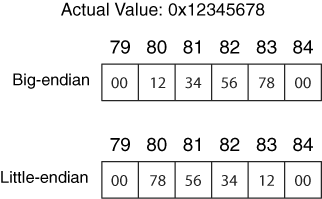IA32-based systems (i.e., Intel Pentium) and their 64-bit counterparts use the little-endian ordering, so we need to "rearrange" the bytes if we want the most significant byte to be the left-most number. Sun SPARC and Motorola PowerPC (i.e., Apple computers) systems use big-endian ordering.

Strings and Character Encoding

The previous section examined how a computer stores numbers, but we must now consider how it stores letters and sentences. The most common technique is to encode the characters using ASCII or Unicode. ASCII is simpler, so we will start there. ASCII assigns a numerical value to the characters in American English. For example, the letter 'A' is equal to 0x41, and '&' is equal to 0x26. The largest defined value is 0x7E, which means that 1 byte can be used to store each character. There are many values that are defined as control characters and are not printable, such the 0x07 bell sound. Table 2.2 shows the hexadecimal number to ASCII character conversion table. A more detailed ASCII table can be found at http://www.asciitable.com/.

 00 NULL 10 DLE 20 SPC 30 0 40 @ 50 P 60 ` 70 p 01 SOH 11 DC1 21 ! 31 1 41 A 51 Q 61 a 71 q 02 STX 12 DC2 22 " 32 2 42 B 52 R 62 b 72 r 03 ETX 13 DC3 23 # 33 3 43 C 53 S 63 c 73 s 04 EOT 14 DC4 24 \$ 34 4 44 D 54 T 64 d 74 t 05 ENQ 15 NAK 25 % 35 5 45 E 55 U 65 e 75 u 06 ACK 16 SYN 26 & 36 6 46 F 56 V 66 f 76 v 07 BEL 17 ETB 27 ' 37 7 47 G 57 W 67 g 77 w 08 BS 18 CAN 28 ( 38 8 48 H 58 X 68 h 78 x 09 TAB 19 EM 29 ) 39 9 49 I 59 Y 69 i 79 y 0A LF 1A SUB 2A * 3A ; 4A J 5A Z 6A j 7A z 0B BT 1B ESC 2B + 3B ; 4B K 5B [ 6B k 7B { 0C FF 1C FS 2C , 3C < 4C L 5C 6C l 7C | 0D CR 1D GS 2D - 3D = 4D M 5D ] 6D m 7D } 0E SO 1E RS 2E . 3E > 4E N 5E ^ 6E n 7E ~ 0F SI 1F US 2F / 3F ? 4F O 5F _ 6F o 7F

To store a sentence or a word using ASCII, we need to allocate as many bytes as there are characters in the sentence or word. Each byte stores the value of a character. The endian ordering of a system does not play a role in how the characters are stored because these are separate 1-byte values. Therefore, the first character in the word or sentence is always in the first allocated byte. The series of bytes in a word or sentence is called a string. Many times, the string ends with the NULL symbol, which is 0x00. Figure 2.6 shows an example string stored in ASCII. The string has 10 symbols in it and is NULL terminated so it has allocated 11 bytes starting at byte 64.

Figure 2.6. An address that is represented in ASCII starting at memory address 64.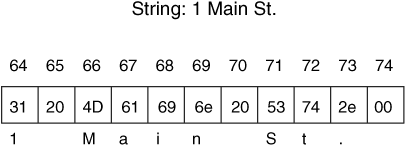ASCII is nice and simple if you use American English, but it is quite limited for the rest of the world because their native symbols cannot be represented. Unicode helps solve this problem by using more than 1 byte to store the numerical version of a symbol. (More information can be found at www.unicode.org.) The version 4.0 Unicode standard supports over 96,000 characters, which requires 4-bytes per character instead of the 1 byte that ASCII requires.

There are three ways of storing a Unicode character. The first method, UTF-32, uses a 4-byte value for each character, which might waste a lot of space. The second method, UTF-16, stores the most heavily used characters in a 2-byte value and the lesser-used characters in a 4-byte value. Therefore, on average this uses less space than UTF-32. The third method is called UTF-8, and it uses 1, 2, or 4 bytes to store a character. Each character requires a different number of bytes, and the most frequently used bytes use only 1 byte.

UTF-8 and UTF-16 use a variable number of bytes to store each character and, therefore, make processing the data more difficult. UTF-8 is frequently used because it has the least amount of wasted space and because ASCII is a subset of it. A UTF-8 string that has only the characters in ASCII uses only 1 byte per character and has the same values as the equivalent ASCII string.

Data Structures

Before we can look at how data are stored in specific file systems, we need to look at the general concept of data organization. Let's return back to the previous example where we compared digital data sizes to boxes on a paper form. With a paper form, a label precedes the boxes and tells you that the boxes are for the name or address. Computers do not, generally, precede file system data with a label. Instead, they simply know that the first 32 bytes are for a person's name and the next 32 bytes are for the street name, for example.

Computers know the layout of the data because of data structures. A data structure describes how data are laid out. It works like a template or map. The data structure is broken up into fields, and each field has a size and name, although this information is not saved with the data. For example, our data structure could define the first field to be called 'number' and have a length of 2 bytes. It is used to store the house number in our address. Immediately after the 'number' field is the 'street' field and with a length of 30 bytes. We can see this layout in Table 2.3.

Table 2.3. A basic data structure for the house number and street name.

Byte Range

Description

01

2-byte house number

231

30-byte ASCII street name

If we want to write data to a storage device, we refer to the appropriate data structure to determine where each value should be written. For example, if we want to store the address 1 Main St., we would first break the address up into the number and name. We would write the number 1 to bytes 0 to 1 of our storage space and then write "Main St." in bytes 2 to 9 by determining what the ASCII values are for each character. The remaining bytes can be set to 0 since we do not need them. In this case, we allocated 32 bytes of storage space, and it can be any where in the device. The byte offsets are relative to the start of the space we were allocated. Keep in mind that the order of the bytes in the house number depends on the endian ordering of the computer.

When we want to read data from the storage device, we determine where the data starts and then refer to its data structure to find out where the needed values are. For example, let's read the data we just wrote. We learn where it starts in the storage device and then apply our data structure template. Here is the output of a tool that reads the raw data.

```
0000000: 0100 4d61 696e 2053 742e 0000 0000 0000 ..Main St.......

0000016: 0000 0000 0000 0000 0000 0000 0000 0000 ................

0000032: 1900 536f 7574 6820 5374 2e00 0000 0000 ..South St......

0000048: 0000 0000 0000 0000 0000 0000 0000 0000 ................

```

The previous output is from the xxd Unix tool and is similar to a graphical hex-editor tool. The left column is the byte offset of the row in decimal, the 8 middle columns are 16 bytes of the data in hexadecimal, and the last column is the ASCII equivalent of the data. A '.' exists where there is no printable ASCII character for the value. Remember that each hexadecimal symbol represents 4 bits, so a byte needs 2 hexadecimal symbols.

We look up the layout of our data structure and see that each address is 32 bytes, so the first address is in bytes 0 to 31. Bytes 0 to 1 should be the 2 byte number field, and bytes 2 to 31 should be the street name. Bytes 0 to 1 show us the value 0x0100. The data are from an Intel system, which is little-endian, and we will therefore have to switch the order of the 0x01 and the 0x00 to produce 0x0001. When we convert this to decimal we get the number 1.

The second field in the data structure is in bytes 2 to 31 and is an ASCII string, which is not effected by the endian ordering of the system, so we do not have to reorder the bytes. We can either convert each byte to its ASCII equivalent or, in this case, cheat and look on the right column to see "Main St.." This is the value we previously wrote. We see that another address data structure starts in byte 32 and extends until byte 63. You can process it as an exercise (it is for 25 South St).

Obviously, the data structures used by a file system will not be storing street addresses, but they rely on the same basic concepts. For example, the first sector of the file system typically contains a large data structure that has dozens of fields in it and we need to read it and know that the size of the file system is given in bytes 32 to 35. Many file systems have several large data structures that are used in multiple places.

Flag Values

There is one last data type that I want to discuss before we look at actual data structures, and it is a flag. Some data are used to identify if something exists, which can be represented with either a 1 or a 0. An example could be whether a partition is bootable or not. One method of storing this information is to allocate a full byte for it and save the 0 or 1 value. This wastes a lot of space, though, because only 1 bit is needed, yet 8 bits are allocated. A more efficient method is to pack several of these binary conditions into one value. Each bit in the value corresponds to a feature or option. These are frequently called flags because each bit flags whether a condition is true. To read a flag value, we need to convert the number to binary and then examine each bit. If the bit is 1, the flag is set.

Let's look at an example by making our previous street address data structure a little more complex. The original data structure had a field for the house number and a field for the street name. Now, we will add an optional 16-byte city name after the street field. Because the city name is optional, we need a flag to identify if it exists or not. The flag is in byte 31 and bit 0 is set when the city exists (i.e., 0000 0001). When the city exists, the data structure is 48 bytes instead of 32. The new data structure is shown in Table 2.4.

Table 2.4. A data structure with a flag value.

Byte Range

Description

01

2-byte house number

230

29-byte ASCII street name

3131

Flags

3247

16-byte ASCII city name (if flag is set)

Here is sample data that was written to disk using this data structure:

```
0000000: 0100 4d61 696e 2053 742e 0000 0000 0000 ..Main St.......

0000016: 0000 0000 0000 0000 0000 0000 0000 0061 ...............a

0000032: 426f 7374 6f6e 0000 0000 0000 0000 0000 Boston..........

0000048: 1800 536f 7574 6820 5374 2e00 0000 0000 ..South St......

0000064: 0000 0000 0000 0000 0000 0000 0000 0060 ...............`

```

On the first line, we see the same data as the previous example. The address is 1 Main St, and the flag value in byte 31 has a value of 0x61. The flag is only 1 byte in size, so we do not have to worry about the endian ordering. We need to look at this value in binary, so we use the lookup table previously given in Table 2.1 and convert the values 0x6 and 0x1 to the binary value 0110 0001. We see that the least significant bit is set, which is the flag for the city. The other bits are for other flag values, such as identifying this address as a business address. Based on the flag, we know that bytes 32 to 47 contain the city name, which is "Boston." The next data structure starts at byte 48, and its flag field is in byte 79. Its value is 0x60, and the city flag is not set. Therefore, the third data structure would start at byte 80.

We will see flag values through out file system data structures. They are used to show which features are enabled, which permissions are in effect, and if the file system is in a clean state.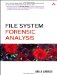File System Forensic Analysis
ISBN: 0321268172
EAN: 2147483647
Year: 2006
Pages: 184
Authors: Brian Carrier